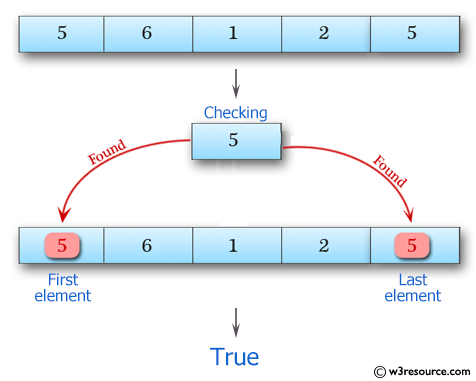﻿ Swift Array Programming Exercise: Check if 5 appears as either the first or last element in a given array of integers - w3resource# Swift Array Programming Exercises: Check if 5 appears as either the first or last element in a given array of integers

## Swift Array Programming: Exercise-1 with Solution

Write a Swift program to check if 5 appears as either the first or last element in a given array of integers. The array length should be 1 or more.

Pictorial Presentation:Sample Solution:

Swift Code:

``````func first_last_5( _ arra:[Int]) -> Bool {
if arra.first == 5 || arra.last == 5
{
return true
}
else
{
return false
}
}
print(first_last_5([1, 2, 5]))
print(first_last_5([5, 1, 2, 3, 4]))
print(first_last_5([5, 6, 1, 2, 5]))
print(first_last_5([1, 2, 6, 5, 3, 7]))
```
```

Sample Output:

```true
true
true
false
```

Swift Programming Code Editor:

Improve this sample solution and post your code through Disqus

What is the difficulty level of this exercise?

﻿